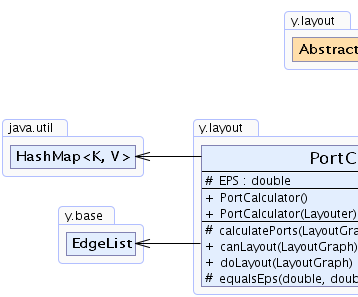Search this API

## y.layout Class PortCalculator

```java.lang.Objecty.layout.AbstractLayoutStagey.layout.PortCalculator
```
All Implemented Interfaces:
Layouter, LayoutStage

`public class PortCalculatorextends AbstractLayoutStageimplements LayoutStage`

A `PortCalculator` can be applied to move ports from the nodes' rectangular bounds to the actual outline.

Layout algorithms only consider rectangular nodes even though the actual shape of a node is, for example, circular. Hence, without adding specific strong `PortConstraint`s, the ports are usually placed at the border of the nodes' `bounding boxes` (except for some layout algorithms that produce straight-line edge routes and place the ports at the nodes' center). A `PortCalculator` may wrap a `core layout algorithm` to adjust the port assignment after the layout calculation. For the calculation of the new port offsets, the `PortCalculator` delegates to instances of interface `IntersectionCalculator`. To specify such an instance for the source/target of an edge, use a `DataProvider` that is registered to the graph with key `IntersectionCalculator.SOURCE_INTERSECTION_CALCULATOR_DPKEY` and `IntersectionCalculator.TARGET_INTERSECTION_CALCULATOR_DPKEY`, respectively. Note that the intersection point (i.e., port) of an edge and the associated source/target node provided by method `IntersectionCalculator.calculateIntersectionPoint(NodeLayout, double, double, double, double)` will be added to the edge's `EdgeLayout`.

This class will not change the coordinates of a port if it is associated with a strong port constraint.
`IntersectionCalculator`, `IntersectionCalculator.SOURCE_INTERSECTION_CALCULATOR_DPKEY`, `IntersectionCalculator.TARGET_INTERSECTION_CALCULATOR_DPKEY`, `PortConstraint`Field Summary
`protected  double` `EPS`
EPSILON used by `equalsEps(double, double, double, double)`.

Fields inherited from interface y.layout.Layouter
`EDGE_ID_DPKEY, NODE_ID_DPKEY, SELECTED_EDGES, SELECTED_NODES`

Constructor Summary
`PortCalculator()`
Creates a new `PortCalculator` instance with default settings.
`PortCalculator(Layouter coreLayouter)`
Creates a new `PortCalculator` instance using the given `core layout algorithm`.

Method Summary
`protected  void` `calculatePorts(LayoutGraph graph)`
Performs the actual port calculation on the specified graph instance.
` boolean` `canLayout(LayoutGraph graph)`
Accepts all graphs that can be handled by the `core layout algorithm`.
` void` `doLayout(LayoutGraph graph)`
Adjusts the ports after invoking the `core layout algorithm`.
`protected  boolean` ```equalsEps(double x1, double y1, double x2, double y2)```
Determines whether two points are equal.

Methods inherited from class y.layout.AbstractLayoutStage
`canLayoutCore, doLayoutCore, getCoreLayouter, setCoreLayouter`

Methods inherited from class java.lang.Object
`clone, equals, finalize, getClass, hashCode, notify, notifyAll, toString, wait, wait, wait`

Methods inherited from interface y.layout.LayoutStage
`getCoreLayouter, setCoreLayouter`

Field Detail

### EPS

`protected double EPS`
EPSILON used by `equalsEps(double, double, double, double)`. By default this is `0.2d`.

Constructor Detail

### PortCalculator

`public PortCalculator()`
Creates a new `PortCalculator` instance with default settings.

### PortCalculator

`public PortCalculator(Layouter coreLayouter)`
Creates a new `PortCalculator` instance using the given `core layout algorithm`.

Parameters:
`coreLayouter` - the core layout routine
Method Detail

### canLayout

`public boolean canLayout(LayoutGraph graph)`
Accepts all graphs that can be handled by the `core layout algorithm`.

If there is no `core layout algorithm`, all graphs are accepted.

Specified by:
`canLayout` in interface `Layouter`
Parameters:
`graph` - the input graph
Returns:
`true` if there is no core layout algorithm, the graph is `null` or the core layout algorithm accepts the graph, `false` otherwise
`Layouter.doLayout(LayoutGraph)`

### doLayout

`public void doLayout(LayoutGraph graph)`
Adjusts the ports after invoking the `core layout algorithm`.

Specified by:
`doLayout` in interface `Layouter`
Parameters:
`graph` - the input graph
`Layouter.canLayout(LayoutGraph)`

### calculatePorts

`protected void calculatePorts(LayoutGraph graph)`
Performs the actual port calculation on the specified graph instance.

Parameters:
`graph` - the input graph

### equalsEps

```protected boolean equalsEps(double x1,
double y1,
double x2,
double y2)```
Determines whether two points are equal.

This implementation uses `EPS` to add some tolerance to the comparison. It is called by `calculatePorts(LayoutGraph)` and may be overridden to change the accuracy/tolerance of the comparison.

Parameters:
`x1` - the x-coordinate of the first point
`y1` - the y-coordinate of the first point
`x2` - the x-coordinate of the second point
`y2` - the y-coordinate of the second point
Returns:
`true` if both points are considered equal, `false` otherwise
`calculatePorts(LayoutGraph)`, `EPS`